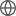Old Web
English
In this study, we use the SWIFT/BAT AGN sample, which has received extensive multiwavelength follow-up analysis as a result of the BAT AGN Spectroscopic Survey (BASS), to develop a diagnostic for nuclear obscuration by examining the relationship between the line-of-sight column densities ($N_{\rm{H}}$), the 2-10 keV-to-$12\,\rm{\mu m}$ luminosity ratio, and WISE mid-infrared colors. We demonstrate that heavily obscured AGNs tend to exhibit both preferentially ''redder'' mid-infrared colors and lower values of $L_{\rm{X,\,Obs.}}$/$L_{12\,\rm{\mu m}}$ than less obscured AGNs, and we derive expressions relating $N_{\rm{H}}$ to the $L_{\rm{X,\,Obs.}}$/$L_{12\,\rm{\mu m}}$ and $L_{22\,\rm{\mu m}}$/$L_{4.6\,\rm{\mu m}}$ luminosity ratios as well as develop diagnostic criteria using these ratios. Our diagnostic regions yield samples that are $\gtrsim80$% complete and $\gtrsim60$% pure for AGNs with log($N_{\rm{H}})\geq24$, as well as $\gtrsim85$% pure for AGNs with $\rm{log}(N_{\rm{H}})\gtrsim23.5$. We find that these diagnostics cannot be used to differentiate between optically star forming galaxies and active galaxies. Further, mid-IR contributions from host galaxies that dominate the observed $12~\rm{\mu m}$ emission can lead to larger apparent X-ray deficits and redder mid-IR colors than the AGNs would intrinsically exhibit, though this effect helps to better separate less obscured and more obscured AGNs. Finally, we test our diagnostics on two catalogs of AGNs and infrared galaxies, including the XMM-Newton XXL-N field, and we identify several known Compton-thick AGNs as well as a handful of candidate heavily obscured AGNs based upon our proposed obscuration diagnostics.